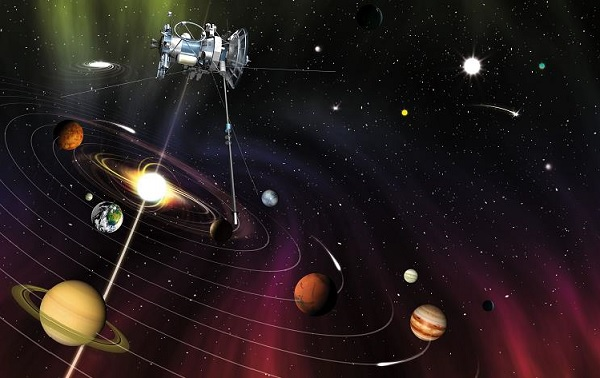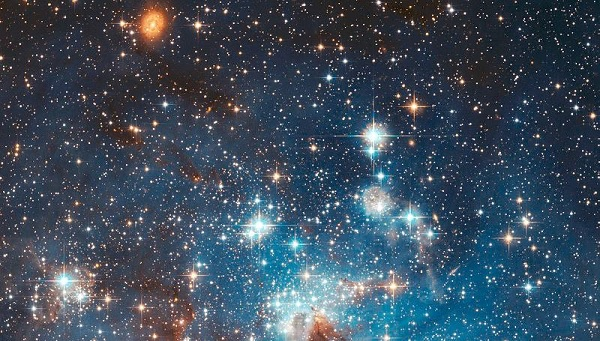# Physics - Astrophysics

## Introduction

• Astrophysics is one of the oldest branches of natural science or astronomy.

• Astrophysics is being used as the basis for making calendars and navigation.

• Astrophysics is also being used as an important input for religions because since the beginning, astrologers taking help of this science to in their astrological works.• The modern branch of astrophysics namely ‘Theoretical astrophysics,’ describes the functions and behaviors of celestial bodies.

• Theoretical astrophysics uses a wide variety of tools such as analytical models (e.g., polytropes to approximate the behaviors of a star) and the computational numerical simulations.

## Topics of Astrophysics

• Following are the major topics of astrophysics (modern) −

• Solar System (formation and evolution);

• Stellar dynamics and evolution;

• Galaxy formation and evolution;

• Magneto-hydrodynamics;

• Origin of cosmic rays;

• General relativity and physical cosmology.

## Major Works in Astrophysics

• Following are the major developments in Astrophysics −

• By using telescope, Galileo had performed the first astronomical studies in 1609. Galileo discovered sun spots and four satellites of Saturn.

• Based on observations of Tycho Brahe, Kepler had developed three laws of planetary motions.

• In 1687, Newton had introduced the laws of motion and gravitation.

• By giving the theory of relativity in 1916, Einstein provided the first consistent basis to study cosmology.

• In 1926, Hubble discovered that the galaxies are recessing and their velocity is increasing with the distance. It means, the universe is expanding and extrapolating this expansion back in time led to the concept of ‘Big Bang.’

• In 1974, Hulse and Taylor discovered a binary system of two pulsars that proved the existence of gravitational waves.

## Astronomy

• Astronomy the oldest branch of is a natural science that studies celestial objects their functional phenomena.

• In order to explain the origin of the celestial bodies, their evolution, and phenomena, the different disciplines of science such as physics, chemistry, mathematics are applied.

• The objects of study are −

• Planets

• Satellites or moons

• Stars

• Galaxies

• Comets, etc.

• Some of the important phenomena those are studied are −

• Supernova explosions

• Gamma ray bursts, and

• Cosmic microwave background radiation, etc.• During the 20th century, based on approach of study, the astronomy is categorized as −

• Observational astronomy − Based on the approach and methods, observational astronomy scientists observe, collect, and analyze the celestial data. To analyze the data, they use basic principles of physics.

• Theoretical astronomy − The scientists of theoretical astronomy attempt to develop computer or analytical models in order to describe the celestial bodies and their functionalities.

• Likewise, astronomy incorporates the diverse disciplines such as celestial navigation, astrometry, observational astronomy, etc.; this is how astrophysics is profoundly related to astronomy.